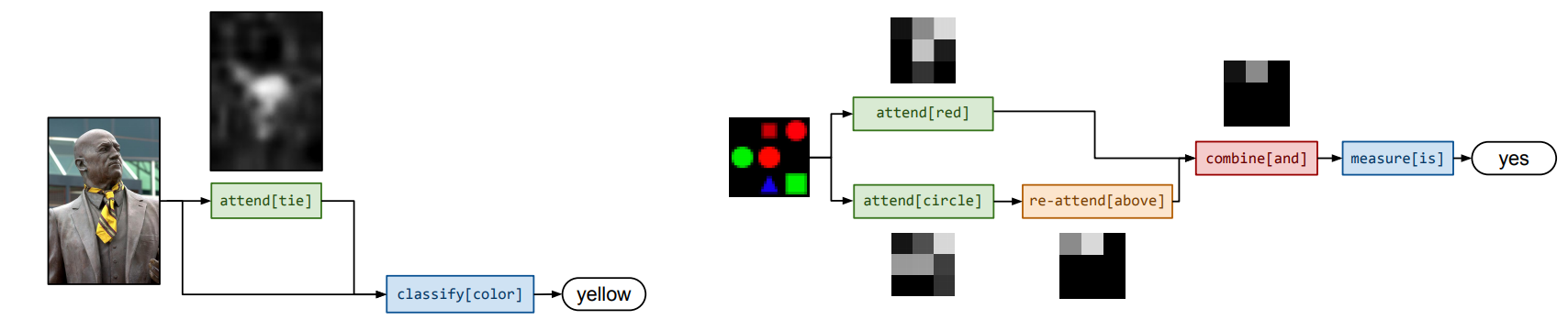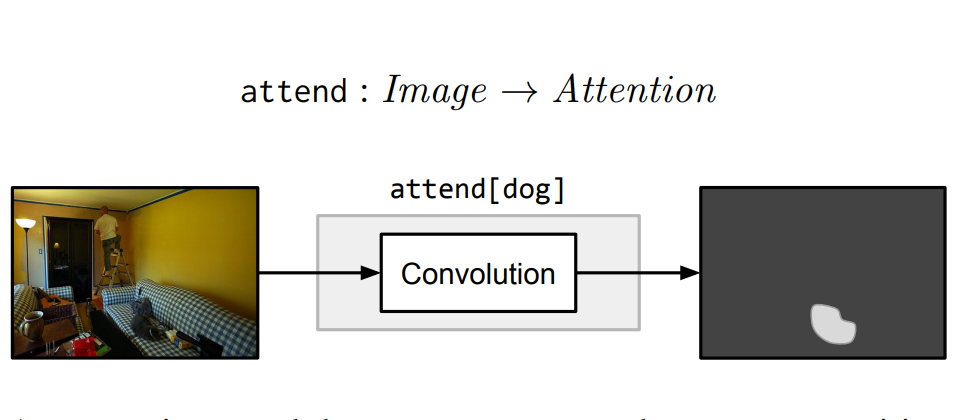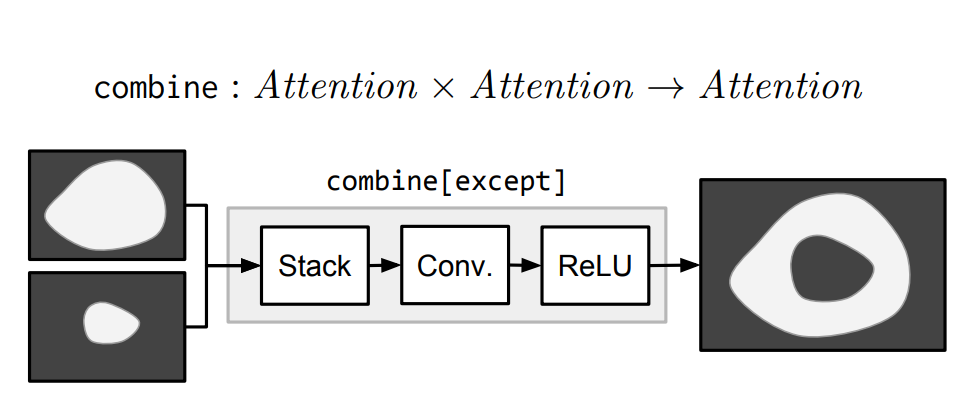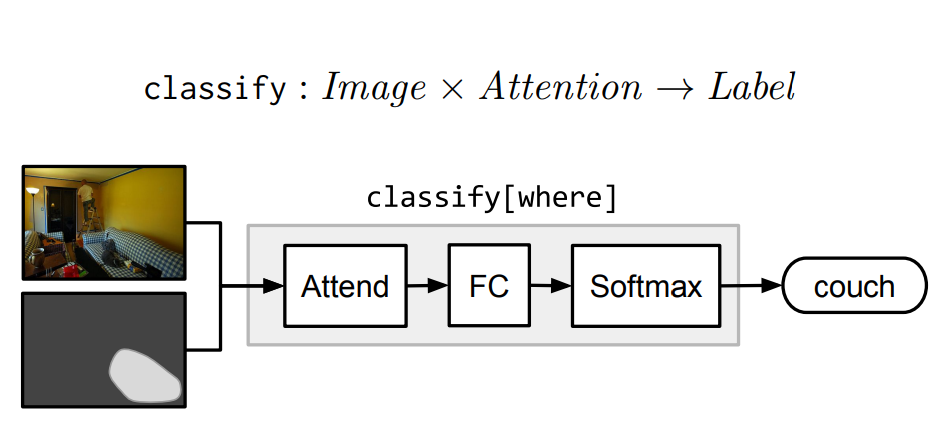# Neural Module Networks实验笔记及总结

2017-08-19-NMN实验笔记及总结# 背景

Visual question answering(VQA)是一个新的数据集，该数据集包含图片及关于图片的一些非定式的问题。这些问题需要计算机结合常识及视觉，语言的理解作出回答。而本文开头介绍的两篇论文介绍是Jacob Andreas提出的对VQA任务所提出的新型模型Neural Module Network（NMN）。后者在前者的基础上作出了改进。

# Neural Module Network• w 是自然语言问题
• x 是图像
• y 是答案

## 模块

### Attentionattend模块将输入图像的每一个位置与与权重（根据C的不同而不同）提供一个热力图或一个非标准的注意力图。比如，attend[dog]模块输出的矩阵，包含狗的区域值较大，而其他区域值较小。

### Re-attentionRe-attention模块本质上由多元感知器及Relu实现，执行一个全连接使得将注意力映射到其他地方。re-attend[above]就是讲attention和最佳的软激活区域向上移。

### Combinationcombine[c]模块将两个attention结合成一个attention。比如，combine[and]只激活两个输入中都激活的区域，而except则是激活第一个输入，将第二个输入失活。

### ClassificationClassification模块classify[c]将attention和image映射到labels的概率分布。它首先计算由注意力加权的平均图像特征，然后通过一个完全连通层传递这个平均特征向量。

### MeasurementMeasure模块Measure[c]以一个attention作为输入，映射到label的概率分布。由于传递的attention是非标准的，所以measure模块适合用于评价检测目标是否存在。

## 网络结构的生成

1. 将自然语言问题映射成布局（layouts）
2. 使用布局(layouts)组建最终的预测网络

### Layout

1. 因为经过简化后，问题的语法等可能影响答案的信息被丢弃了。比如 what is flying? 和 what are flying？ 都变成了 what(fly)。但回答却可能是kite和kites。问题编码器让我们学习数据中的潜在语法规则。
2. 允许我们学习常识性知识。当图片确实或者模糊时，模型可以回答不至于太离谱。

# Learning to Compose Neural Networks for Question Answering

• 首先是对各个模块上进行改进。
• 本文在网络布局模块中加入了增强学习，动态的对每个实例的网络结构进行学习。

1. w: 图像
2. x: 问题
3. y: 答案
4. z: 网络布局
5. $\theta$: 模型参数集合

D-NMN基于两个分布：一个是layout model $p(z|x;\theta_l)$，该分布用于为句子选取布局。另一个是execution model $p_z(y|w;\theta_e)$，在z布局的条件下，对w问题的答案分布。

## 模型

D-NMN更新了新的模块及其实现方式：

### lookup $(\rightarrow Attention)$

lookup[i] 根据words和positions之间的映射关系$f$生成attention。

$\big[lookup[i]\big] = e_{f(i)}$

$e_i$单位向量，$i$th所在的postion为1，其他都为0.

### Find $(\rightarrow Attention)$

find[i]通过每个输入的feature的postion计算每个索引的分布：

$\big[Find[i]\big] = softmax(a \odot \sigma(Bv^i \oplus CW \oplus d ))$

### Relata $(Attention \rightarrow Attention)$

relate 将attention从一个区域转移到另一个区域，它和Find模块很像， 但在当前区域的attention h上增加了条件因素。$\overline{w}(h) = \sum_k{h_kw^k}$

$\big[relate[i](h)\big] = softmax(a \odot \sigma(Bv^i \oplus CW \oplus D\overline{w}(h) \oplus e ))$

### And $(Attention^* \rightarrow Attention)$

and 对输入的attentions做交集操作。

$\big[and(h^1, h^2, ...)\big] = h^1 \odot h^2 \odot ...$

### Desrible $(Attention \rightarrow Lables)$

describe[i] 计算输入attention的加权平均值，该平均值用于预测答案：

$\big[describe[i](h)\big] = softmax(A \sigma(B\overline w(h)+v^i))$

### Exists $(Attention \rightarrow Lables)$

$\big[exists(h)\big] = softmax((\max_k h_k)a + b)$

## 布局选择1. 将输入的局则解析成相互依赖的树型结构
2. 收集跟着疑问词或系动词的名词，动词和介词。
3. 将他们映射成布局的片段。比如：普通名词映射为find模块，专有名词映射为lookup模块，介词映射为两层深度的布局，用relate模型和find模型去联合表示。

$s(z_i|x)=a^T\sigma(Bh_q(x)+Cf(z_i)+d)$

$p(z_i|x;\theta_l) = \frac{e^{s(z_i|x)}}{\sum_{j=1}^{n}e^{s(z_j|x)}}$

$\nabla J(\theta_l) = E[\nabla log p(z|x;\theta_l) * r]$

r 为奖励。

$\nabla J(\theta_l) = E[\nabla log p(z|x;\theta_l) * log p(y|z,w;\theta_e)]$

# Code

code需要编译一个第三方改编的caffe——(apollocaffe)[https://github.com/Russell91/apollocaffe]

File "t.py", line 12, in
net.f(Convolution('conv', (1,1), 1, bottoms=['data']))
File "/home/ubuntu/apollocaffe/python/apollocaffe/layers/caffe_layers.py", line 24, in init
super(Convolution, self).init(self, name, kwargs)
File "/home/ubuntu/apollocaffe/python/apollocaffe/layers/layer_headers.py", line 6, in init
self.parse(sublayer, name, kwargs)
File "/home/ubuntu/apollocaffe/python/apollocaffe/layers/layer_headers.py", line 57, in parse
raise AttributeError('Layer %s has no keyword argument %s=%s' % (param_type, k, v))
AttributeError: Layer Convolution has no keyword argument kernel_w=1


python/apollocaffe/layers/layer_helpers.py#L10.

param_names = [s.name for s in layer.DESCRIPTOR.fields if s.name.endswith('_param')]


# 总结

• R. Hu, J. Andreas, M. Rohrbach, T. Darrell, K. Saenko, Learning to Reason: End-to-End Module Networks for Visual Question Answering. in ICCV, 2017. (PDF)

Project Page: http://ronghanghu.com/n2nmn

Written on August 19, 2017